# 令人不悦的Error-TypeError: Cannot perform ‘ror_‘ with a dtyped [...简说Python |171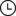2021-09-16 21:03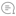0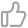00

``# 简单处理数据，去除推荐热搜和置顶热搜wb_hot_data.drop(wb_hot_data[wb_hot_data['wb_rank']=='•' | pd.isna(wb_hot_data['wb_rank'])].index)``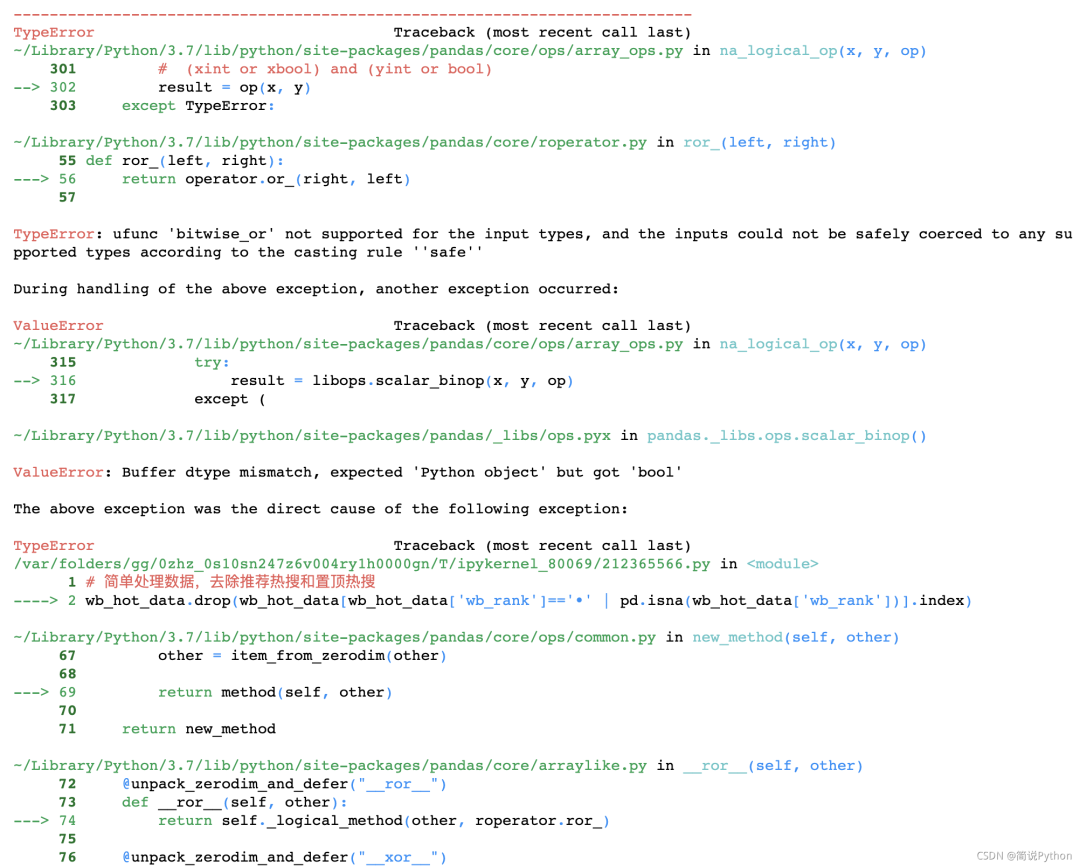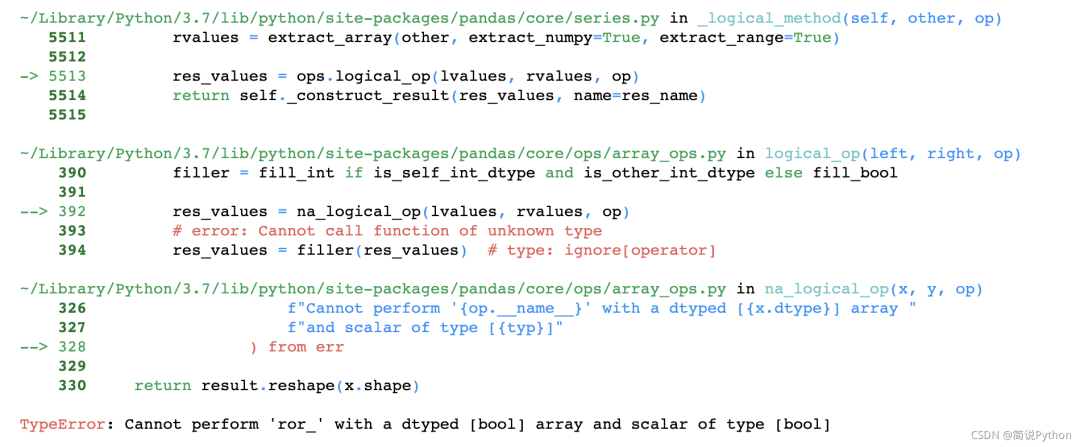看着这一堆报错，我估计代码也很委屈！！！

``# 简单处理数据，去除推荐热搜和置顶热搜wb_hot_data.drop(wb_hot_data[(wb_hot_data['wb_rank']=='•') | pd.isna(wb_hot_data['wb_rank'])].index)``

``import pandas as pdimport numpy as np a=np.array([['a',2,3],['b',5,6],[np.NaN,8,9],['a',4,7]])df1=pd.DataFrame(a,columns=list('ABC'))print(df1)'''  A  B  C0    a  2  31    b  5  62  nan  8  93    a  4  7'''``

``df1.drop(df1[df1['A']=='a' | pd.isna(df1['A'])].index)``

``df1.drop((df1[df1['A']=='a') | pd.isna(df1['A'])].index)# 加个括号就可以啦''' A B C1 b 5 62 nan 8 9'''``

``import pandas as pdimport numpy as np # a=np.array([['a',2,3],['b',5,6],[np.NaN,8,9],['a',4,7]])a=np.array([[1,2,3],[2,5,6],[np.NaN,8,9],[2,4,7]])df1=pd.DataFrame(a,columns=list('ABC'))print(df1)''' A    B    C0  1.0  2.0  3.01  2.0  5.0  6.02  NaN  8.0  9.03  2.0  4.0  7.0'''# 我想删除A列中值为2或者None的行df1.drop(df1[df1['A']==2 | pd.isna(df1['A'])].index)'''A B C1 2.0 5.0 6.02 NaN 8.0 9.03 2.0 4.0 7.0'''``

``df1.drop(df1[(df1['A']==2) | pd.isna(df1['A'])].index)''' A B C0 1.0 2.0 3.0'''# 为什么这里可以把nan值删除？大家可以讨论讨论``

### 参考

 stackoverflow相关问题解答 https://stackoverflow.com/questions/20333435/pandas-comparison-raises-typeerror-cannot-compare-a-dtyped-float64-array-with

 Python运算符优先级和结核性一览表 http://c.biancheng.net/view/2190.html

`--End--文章点赞超过100+我将在个人视频号直播(老表Max)带大家一起进行项目实战复现扫码即可加我微信老表朋友圈经常有赠书/红包福利活动点击上方卡片关注公众号，回复：1024领取最新Python学习资源学习更多：整理了我开始分享学习笔记到现在超过250篇优质文章，涵盖数据分析、爬虫、机器学习等方面，别再说不知道该从哪开始，实战哪里找了“点赞”就是对博主最大的支持`00

暂无评论~~
Ctrl+Enter